﻿ Supersymmetry (SUSY), accelerator is a waste of money.

# Supersymmetry is a waste of money.   Stop it !

## Why "fantasy" Supersymmetry (= SUSY ) is needed ?

(Fig.1) Supersymmetric particles really exist ?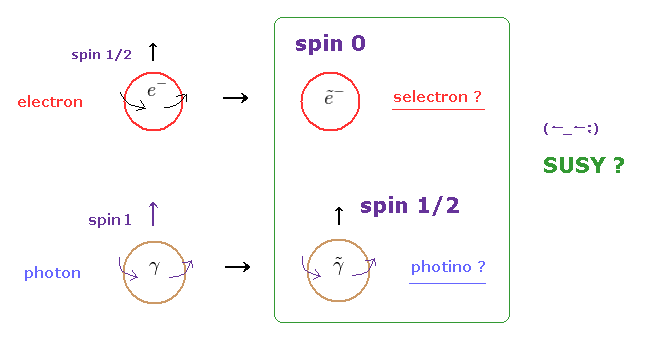According to supersymmetry theory, each elementary particle has its partner.
For example, fermions such as electron and quark have their boson partners, selectron and squark.

As shown in Fig.1 and this site, selectron is a spin 0 boson, and photino (= photon's SUSY ) is a spin 1/2 fermion.
This means the number of elementary particles increases almost two times in SUSY theory !

As you feel, the unified theory must decrease the particle's number.
So supersymmetric theory is very unreasonable.

## Dark matter is really made of "fantasy" SUSY particles ?

(Fig.2) Dark matter is SUSY ?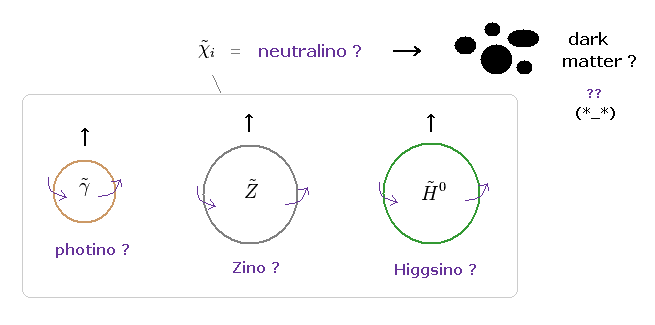All of photino (← photon ), zino (← Z boson ), and Higgsino (← Higgs ) have spin 1/2 and neutral charge.
SUSY insists these are superposition states, which form "neutralino".

If supersymmetry particles were as light as usual elementary particles, they would have been already found in the accelerator.
So they started to argue SUSY particles are very heavy ( ~ 1000 GeV, about 1000 times proton ! ).

In the present theory, dark matter accounts for 30 % of total energies in the universe.
Ordinary neutrino is too light to explain this "heavy" dark matter.
So there are ONLY "unrealistic" SUSY particles such as neutralinos and gravitinos as dark matter's candidate.

## Supersymmetry breaking shows SUSY is "unnecessary".

(Fig.3) "Supersymmetry breaking" is a very convenient concept.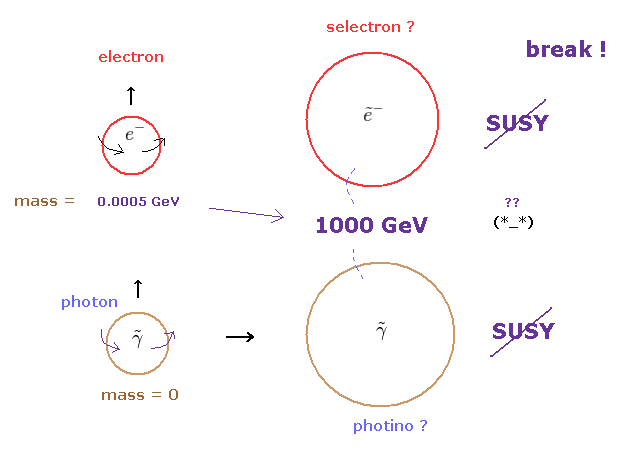Supersymmetry means each elementary particle and its SUSY partner must have the same mass and charge.
But if so, we would have already found SUSY particles around us, which is inconsistent.

So, supersymmetry has to be broken (← very convenient ! ).
If SUSY particle has much bigger mass ( about 1000 GeV ), it cannot be discoverd in our daily life.
But if supersymmetry is broken from the beginning, it means SUSY theory itself is meaningless.

In fact, the standard model needs to depend on very abstract concept such as "symmetry".
Due to the strict limitation of relativity, "artificial" symmetry is the ONLY tool to develop the theory.
This is disaster.

## Almost all elementary particles are "unstable" !?

(Fig.4) Only "electron" is stable ?   Selectron, positron, "spositron" are unstable ?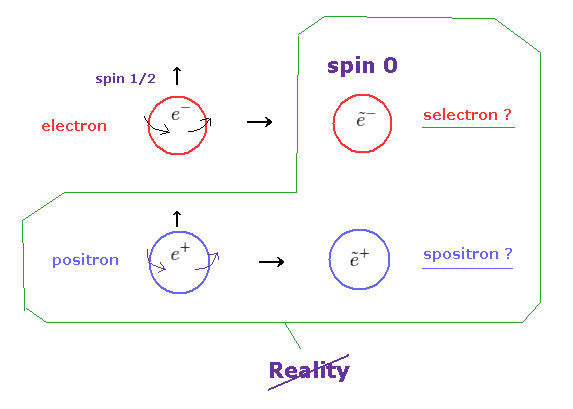If electron's boson partner "selectron" exists, there is "spositron", too.
In all these elementary particles, only "electron" is stable.

As you feel, this SUSY theory introduced too many unnecessary particles.
True "elementary" particles should be very stable for a long time.

## What is spin "0" ?   ← Spin stops ?

(Fig.5) "Spin stopper" = SUSY transformation !?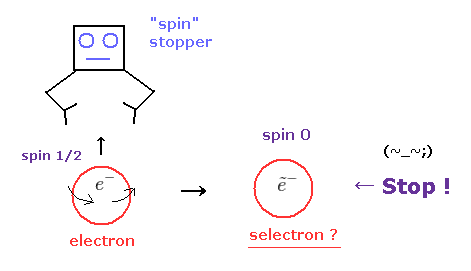It is said that Higgs boson with zero spin was ( indirectly ) found.
This means Higgs particle cannot spin forever ?

If so, what on earth prevents Higgs from rotating ?
This is strange.
If spin zero is real, supersymmetric transformation needs to be performed by some "spin stopper".

Question: Where are these spin stoppers, now ?
Again, this is strange.

## Where does "Spin rotator" fly from ?

(Fig.6) Spin rotator generates Higgsino ?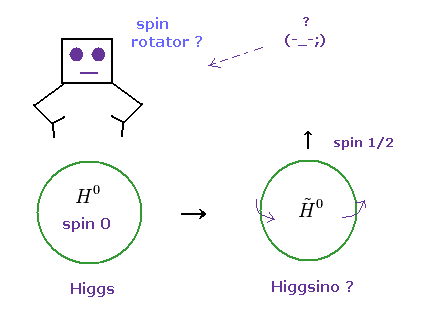According to SUSY, Higgsino has spin 1/2.
Contrary to ordinary fermions, "spin zero" Higgs is more stable than spin 1/2 Higgsino. Why ?

To generate Higgsino, "spin rotator" must fly from somewhere.
We want to know how these spin stopper and rotator are made.

But the current theory can NEVER answer this important question. (= "Shut up and calculate !" )

## Antiparticle of photino does NOT exist ? Too good to be true.

(Fig.7) Antiparticle is a very convenient tool.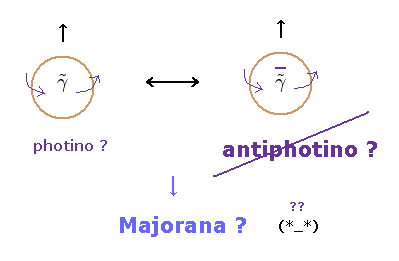Photon is neutral, so its fermion partner photino is neutral, too.
But fermions such as electron and quarks always have their antiparticles.

This means photino has its antipartcile ?
Strange to say, according to SUSY, these photinos are Majorana particles, which means particle and antiparticle are tha same thing.

At this point, you easily find these supersymmetric particles are too good to be true.

## Chiral, gauge symmetries make fermions, photon "massless" !?

(Fig.8) What are "chiral" symmetry and "gauge" symmetry ?   ← Physics ?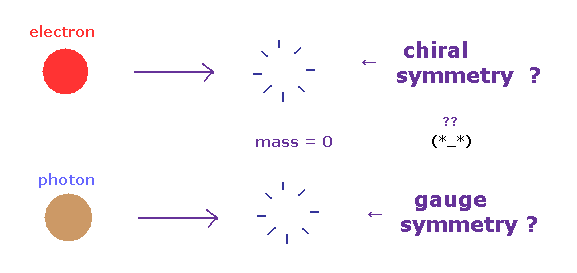According to the standard model, left-handed and right-handed fermions are separated, when their mass is zero.
This is called chiral symmetry ( see this section ).

And due to gauge symmetry, photon's mass becomes zero, they insist.
As you see, the current particle physics blindly believes very abstract math "symmetry".

## Supersymmetry → Higgs mass becomes small !?

(Fig.9) Supersymmetry is needed for "light" Higgs ?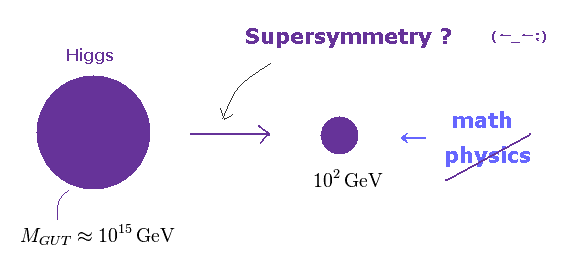As I said above, fermion's mass becomes zero due to chiral symmetry.
Vector boson such as photon becomes massless due to gauge symmetry.

The problem is, there is NO symmetry to describe Higgs small mass ( ~126 GeV ) in the standard model.
So they introduced artificial supersymmetry to make Higgs a light particle. See this site and this site.

Because Higgs is scalar boson with spin zero, which is different from fermions and vector boson (= spin 1 ).
But this reason is NOT physics at all. It's just unsubstantial math symbols.
See this section.

## "Missing energy" → SUSY particle is very "vague" concept.

(Fig.10) SUSY particle cannot be detected directly in the accelerator.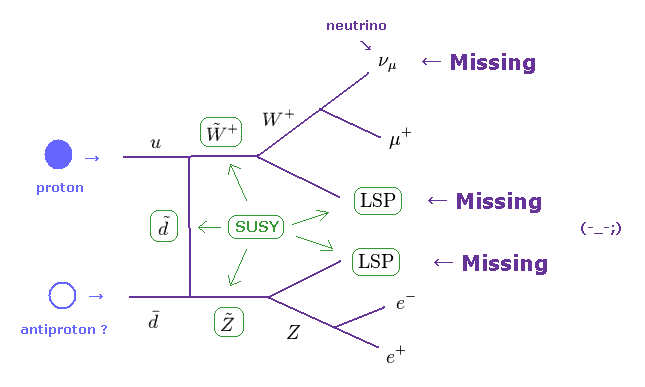The importatn point is that supersymmetry (SUSY) particles cannot be detected directly.
According to R-parity conservation, SUSY particle always decays into SUSY particle.

This means there are some lightest supersymmetric particles (= LSP ), which can be stable forever.
But this LSP leaves NO signature in the detector, like neutrino.

So this presence must be inferred from missing transverse energy.
To know correct missing energy, they have to detect all other scattered energies.
Of course, it is impossible under infinite background.

Besides this vague LSP, ordinary neutrino is missing energy, too.
So the present high energy physics depends on very forced interpretation of experimental results.

## "Higgs" really exists ?

(Fig.11) "Infinite" Higgs are distributed around us, and giving mass ?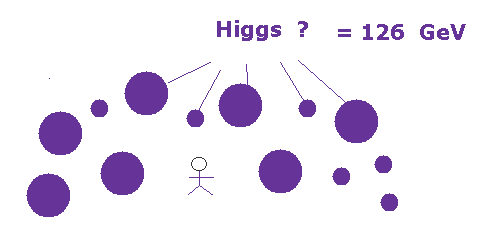According to this site and this site, infinite Higgs particles are uniformly distributed all over the space, and giving mass to every elementary particle.
Ordinary webnews sites often say, "Though Higgs are all around us in the space, it's extremely hard to find them."

As you feel, these expressions are self-contradictory.
If infinite Higgs are distributed all over the space, we can easily find and utilize Higgs for our daily living.

But it's impossible. This is strange.

## Higgs in accelerator is inconsistent with original "infinite" Higgs.

(Fig.12) Only one Higgs appears in "trillions" of collisions !?   ← Really all over the space ?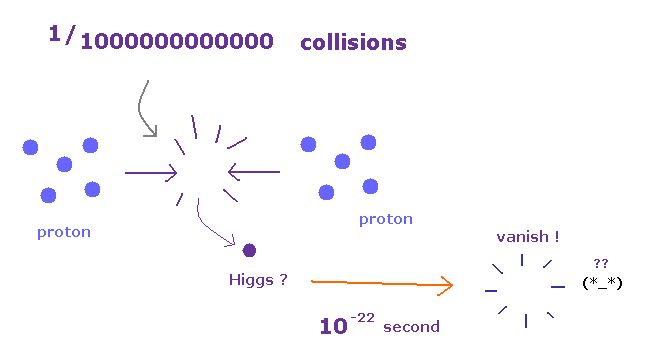This site and this site say more than 1 trillion collisions are necessary to generate one Higgs boson. It's like looking for a needle in a haystack.

When you see these comments, you will probably think, this very rare Higgs inside accelerator is completely inconsistent with the original Higgs, which are distributed all over the space.

For example, electrons are said to form all atoms with nuclei.
Actually, we can easily find electrons by ionizing any atoms or molecules.
So, electrons are consistent with the original definition, which means "real".   But Higgs is NOT.

Futhermore, as shown on this site, Higgs lifetime is only 10-22 seconds ! Very unstable.
So Higgs inside accelerator doesn't satisfy the original definition at all, which means "unreal".

## Top quark is "unrealistic" elementary particle.

(Fig.13) Top quak is as heavy as gold atom !   But elementary particle !?As shown on this site, top quak mass is about 174000 MeV, which is as heavy as gold atom.
In spite of this large mass, the lifetime of top quark is extremely short ( Only 10-25 seconds ! )

According to this site, out of as many as 6 trillion collisions only forty top quarks were observed.
Of course, fractional charges such as +2/3. -1/3 cannot be detected, so quark themselves are just "fictitious" particles.

## 98% of proton's mass is NOT by Higgs mechanism.

(Fig.14) 98% of nuclear mass is due to QCD.   Higgs accounts for only 2% of it.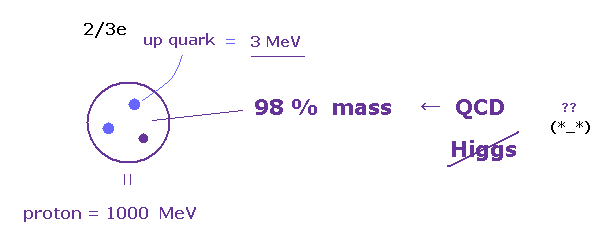As shown on this site, quark's mass acoount for only a few percent of nuclear mass.
More than 98 % of nuclear mass originates in chiral symmetry breaking ( gluon interaction ).

And the masses of "up" and "down" quarks are free parameters ( NOT experimental values ), which can be changed freely.
Very heavy top quark and very light up quarks have the same charge (= +2/3e ) !

But top quark cannot form nuclei, which mass (= gold-like ) is caused only by Higgs, they insist.
Seeing these far-fetched interpretations, you will surely think these quarks are very convenient unrealistic concepts

## W boson in accelerator is completely different from β decay.

(Fig.15) W boson is too heavy (= 80000 MeV ), which cannot be generated from tiny down quark.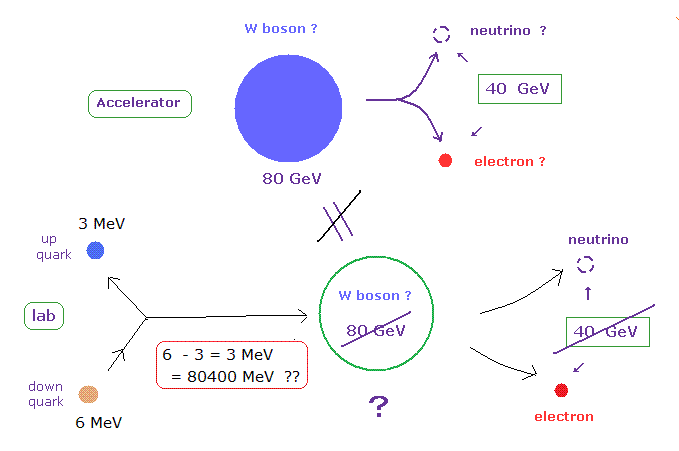The extremely large mass (= 80 GeV is 80 times proton's mass ) of this W boson is gotten from data based on the high energy accelerator.
So this W boson in the accelerator is completely different from that of beta decay in the laboratory.

In usual β decay, down quark inside neutron changes into up quark inside proton emitting negative W boson, they insist.
But the energy difference between down and up quarks is only 3 MeV, which is much smaller than the W boson mass (= 80 GeV = 80000 MeV ). It means W boson in accelerator is a different thing from β decay.

And the lifetime of W boson is only 10-25 seconds, and only ten W bosons were produced from about a billion collisions, as shown on this site.
It is natural to get a few wanted data, if they repeat almost infinite collisions.
So the accelerators did NOT prove Higgs, quarks and W bosons at all.

## Even if they find SUSY, Higgs and quarks, it's completely meaningless.

(Fig.16) Higgs, quarks, and SUSY are found ? → There is NO "next" thing to do.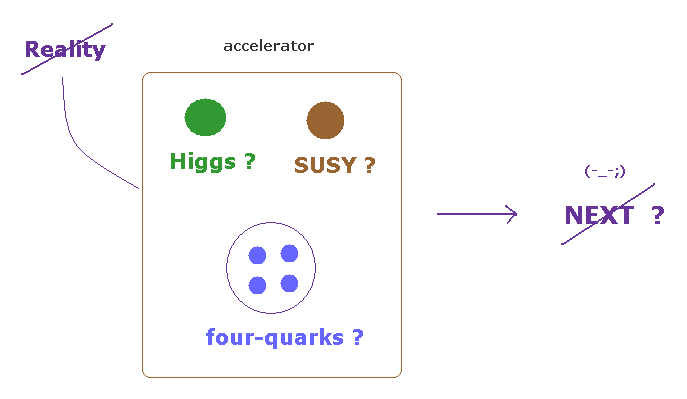Even if physicists argue "Higgs, SUSY and four-quarks " have been found", it's completely meaningless.
These unstable particles cannot be observed directly.
Furthermore, almost infinite collisions are necessary to produce them.

So the hunting for unstable particles in accelerator is very similar to research on early universe and black hole.
Even if we waste a great deal of money and time in these expensive accelerators, we cannot utilize these fictitious particles forever.

First, very rare Higgs is completely inconsistent with the original definition of "infinite" Higgs around us.
Unuseful ( directly and undirectly ) particles means these are unreal.

## Weak isospin, hypercharge are very "artificial" concepts.

(Fig.17) Hypercharge changes ONLY by its moving direction ??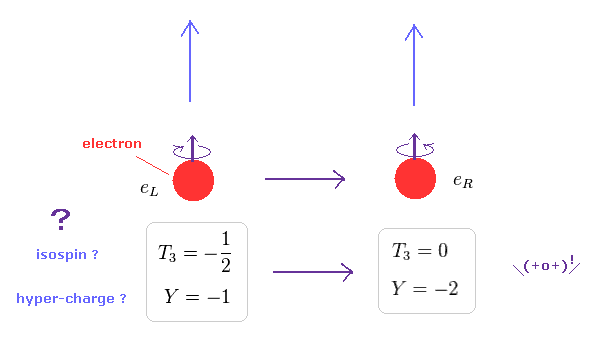As shown on this site, in the right-handed particle, the direction of its spin is the same as the direction of its motion.
In the left-handed, their directions are opposite.

The current standard model considers these very vague helicity as most important.
Surprisingly, weak isospin and hypercharge are completely different between these right and left -handed electrons, as shown on this site.

As you see Fig.17, when an electron changes its moving direction, its hypercharge becomes "-2" from "-1" !
Then, this hypercharge is NOT a fundamental charge !? This is strange.

(Fig.18)   What is "hypercharge" ? → "Shut up and calculate !"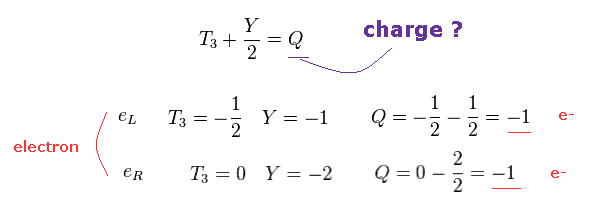Of course, both left-handed (= eL ) and right-handed (= eR ) electrons have one negative charge (= -1 ).
To fit this fact, they just adjust each weak isospin (= T3 ) and hypercharge (= Y ).

So these isospin and hypercharge are very artificial concepts.
And it is unnatural that these hypercharges changes ONLY by its moving direction.
Physicists NEVER try to answer the question of what these hypercharges really are.

## "Antiparticles" conflict with basic principles.

(Fig.19) Positron really exists ?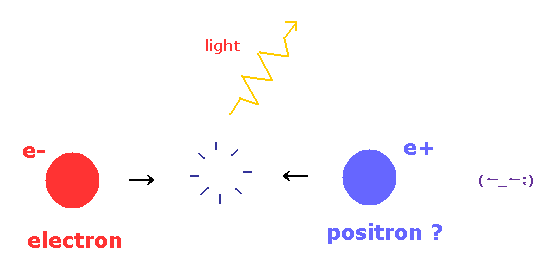Positron is the antiparticle of the electron.
The positron has a opposite charge +e and the same mass as an electron.

According to the current quantum theory, a positron easily annihilates with electron, forming γ rays.
The important point is that the existence of these antiparticles are completely inconsistent with basic physical principles.

This means antiparticles are unreal.

## Why "nucleus" is necessary for pair production from vacuum ?

(Fig.20) Positron is produced from photon.   Why "nucleus" is necessary ?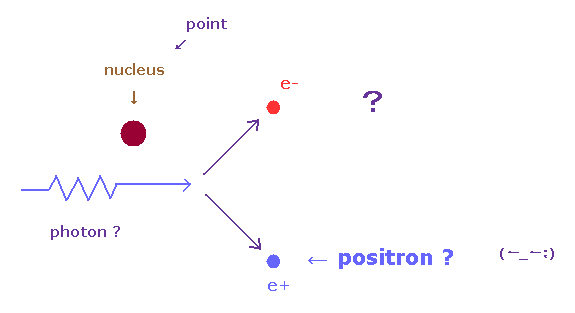According to this site and this site, when a high-energy electron comes near a nucleus, and is deflected in its path, it radiates photons.
These photons spontaneously turn into an electron-positron pair.

But if this explanation is right, the nucleus (= target ) is NOT necessary.
ONLY under the strong electromagnetic field, each electron can radiate high-energy lights !

Then why they must collide electrons with other nuclei to produce positron ?
The reason of this is very unreasonable.

## Momentum (= p ) is NOT conserved in positron production !

(Fig.21) Momentum of incident light suddenly becomes zero ( p = 0 ) !?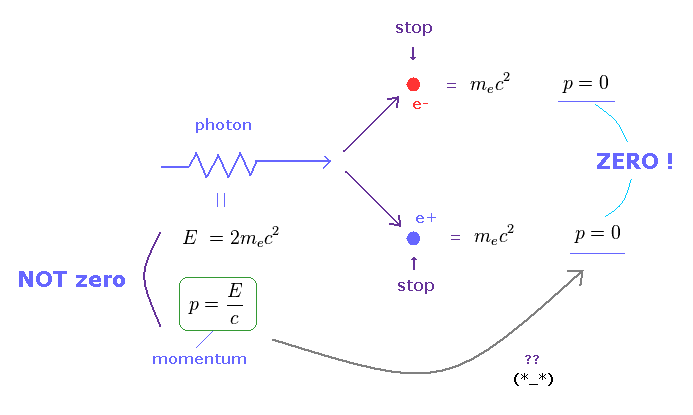This website says,
----------------------------
If the photon only just had enough energy to create the mass of the electron-positron pair then the electron and positron will be at rest.
This could violate the conservation of momentum since the photon has momentum ( p = E/c ) and the two resulting electrons have none ( p = 0 ) if they are stationary ( momentum = mass × velocity ).

This means that the pair production must take place near the nucleus since they can absorb the momentum of the original photon.
-----------------------------

It is impossible for the nucleus to absorb ONLY momentum, without energy.
Because absorbing momentum means absorbing some kinetic energy.

(Fig.22) Only momentum is NOT conserved.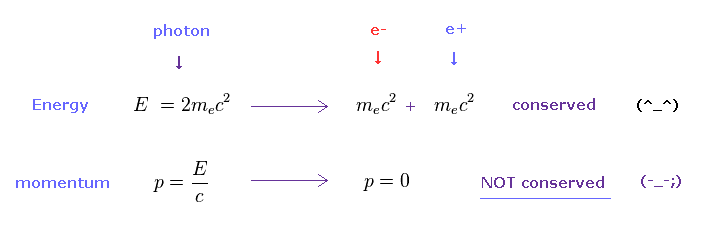As shown in Fig.22, when the incident photon has the energy of just 2mc2, one pair of electron and positron can be created, according to Einstein relation.

But in this case, these resultant electron-positron do NOT have kinetic energy at all.
Because all energies of the photon are used for their mass energy.

Of course, the incident light has momentum ( p = E/c ).
But the resultant electron-positron has NO momentum ( p = 0 ). This is strange.

## "Artificial" nucleus absorbs ONLY momentum, NOT energy !?

(Fig.23) Convenient nucleus can absorb "excessive" momentum ?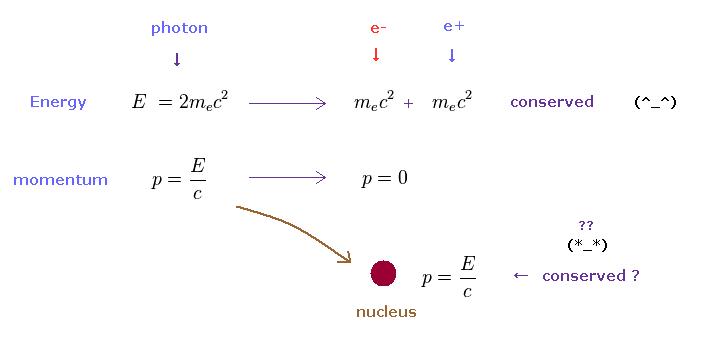Of course, the total momentum ( and energy ) must be conserved.
To be consistent with this basic law, they think the nucleus near photon absorbs this excessive momentum.

As you may notice, this explanation is unreasonable.

(Fig.24) This nucleus has "momentum", but NOT kinetic energy !?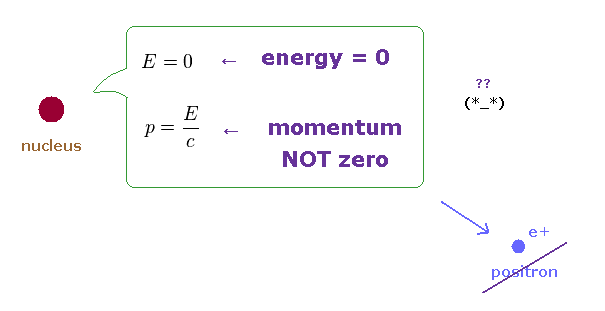Strange to say, this nucleus has momentum, but NO kinetic energy !
In the realistic world, this is impossible.

This fact clearly shows pair production of positron-electron is impossible.
A positron is just a positive ion or proton ( or scattered electrons ), considering it always needs collision with nuclei.

## Positron-electron annihilation is unrealistic, too.

(Fig.25) Positron-electron annihilation is real ?Next we think about the annihilation of electron positron pair.
According to Feynman rule, this pair produces one new photon.

(Fig.26) Center of mass system.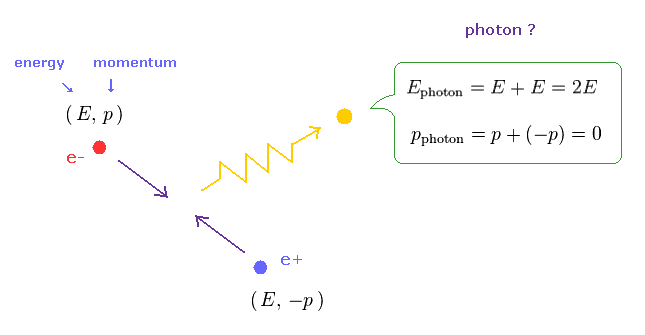We think about the center of mass system, in which electron and positron are flying in the opposite directions, and crash into each other.
According to the energy and momentum conservation, the resultant photon's energy is twice the original particle.

(Fig.27)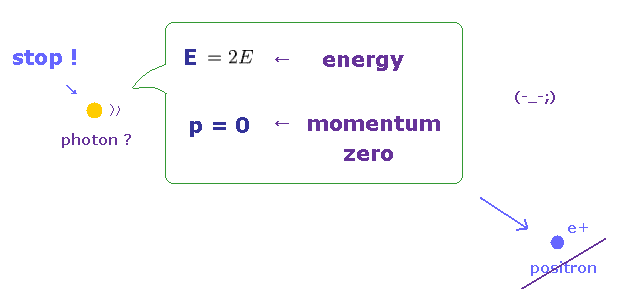But the phoron's momentum is cancelled out to be zero, which means the resultant photon stops ! See this site (p.2).
Of course, photon (= light ) cannot stop, so this photon is virtual photon, which violates relativity.

According to Feynman diagram, first, only one photon is produced by electron-positron annihilation.
As you see, the existence of positron causes self-contradiction in the relativistic quantum field theory.

## β+ decay shows "positron" is illusion.

(Fig.28) Na22 isotope can decay by both electron capture and β+ decay.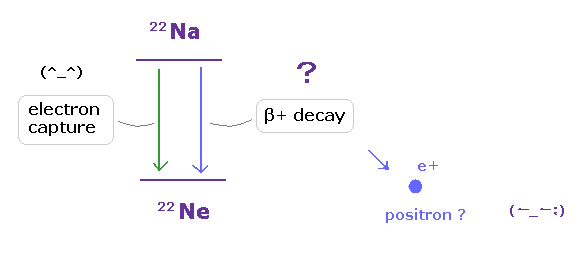As shown on this site and this site (p.3), the decay of Na22 proceeds by positron β+ emission (90%) or electron capture (10%) to pruduce Ne22.

In both β+ decay, and electron capture, the positive charge of Na22 nucleus decreases by one.
But you easily find it is impossible that both these decays happen in the same kind of nuclei.

(Fig.29) Electron capture:   Na nucleus "absorbs" one negative enectron.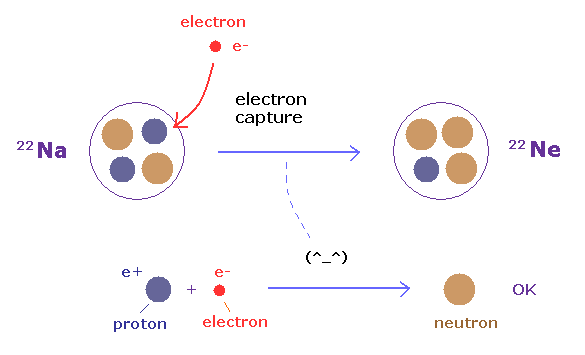In electron capture, nucleus absorbs one negative electron.
So the atomic number of Na (= 11 ) changes into Ne (= 10 ).

Inside Na nucleus, a proton and an electron form one neutron.
A neutron is a little heavier than a proton, so this process is very natural.

(Fig.30) β+ decay:   Na nucleus emits one positron ?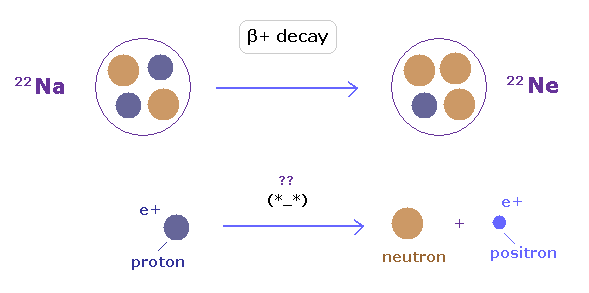In β+ decay, Na nucleus emits one positive positron.
So also in this case, positive charge (= atomic number ) of Na nucleus decreases by one.

This is the reason why they argue both electron capture and β+ decay give the same Ne nucleus.
But as you see, in electron capture, Na nucleus absorbs a particle, on the other hand, in β+ decay, Na nucleus emit a particle !
These are NOT the same.

## β+ decay → Proton's positive charge is removed ?

(Fig.31) A Proton can be divided into two ? → positron ?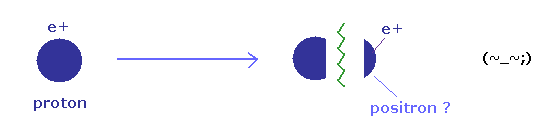As you know, the positive charge of atomic nuclei originates in protons.
Because, very unstable positron cannot stay inside nuclei for a long time.

This means in β+ decay, the protons inside Na nucleus must be divided into two, to give its positive charge to positron !
Of course, this is impossible.

(Fig.32) Where energy is emitted ?   Their places contain self-contradiction.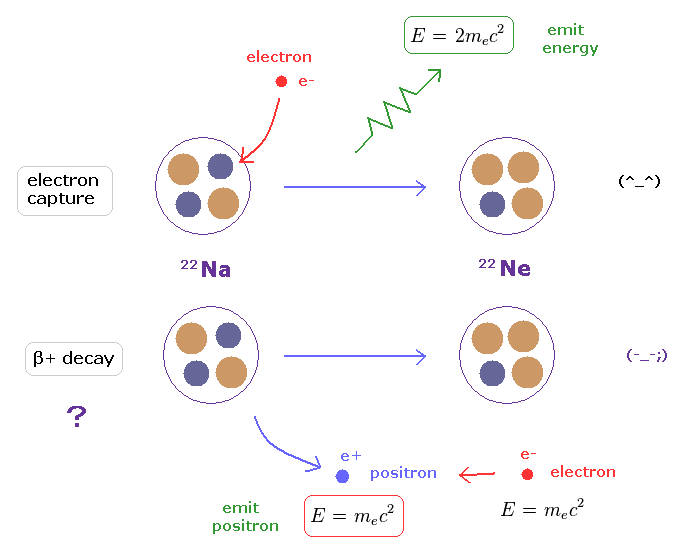The same resultant Ne nucleus means the same amount of energy must be emitted in both electron capture and β+ decay.
In electron capture ( Fig.32 upper ), this energy is emitted from absorbing Na nucleus itself.

On the other hand, in β+ decay ( Fig.32 lower ), Na nuceus just emits a positron instead of energy.
And this positron annihilates with an external electron, and emits the energy of 2me2 somewhere else.

This means a part of emitted energies needs to be teleported to unrelated external electron (= mass energy ) somewhere else, only in β+ decay !
Of course, this is impossible, so β+ decay (= positron ) is unrealistic.

## How is a positron source Na22 produced ?

(Fig.33) Breaking Mg nuclei generates Na22 source. ← Positron is NOT involved at all.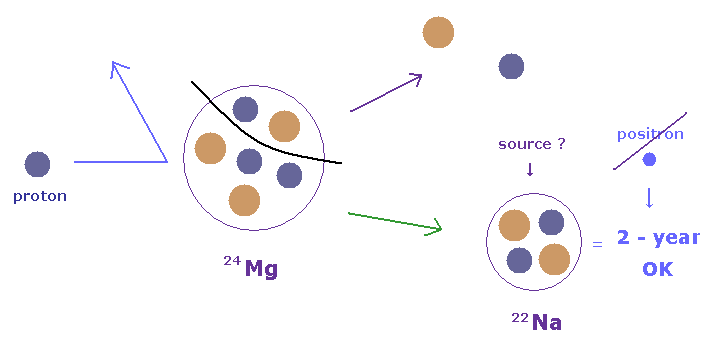As shown on this site, to produce positron source Na22, a magnesium target is bombarded for several weeks with a high-energy proton beam.
This means Na22 nucleus can be produced by removing one proton and neutron from Mg nucleus.

And the lifetime of this Na22 positron source is as long as 2 years.
So very unstable positron cannot stay inside Na nucleus, even if it is produced at the first bombardment.

From this fact, you find Na22 source has Nothing to do with "fantasy" positron.
This Na22 has excessive proton, which tends to absorb electron through electron capture.

## Positron moderator does NOT prove positron.

(Fig.34) Positron moderator can slow down positron ?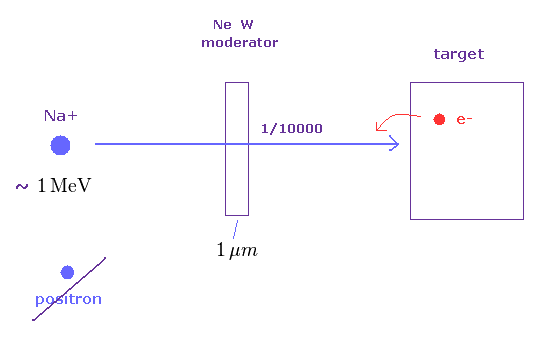As shown on this site and this site, moderator is said to slow high energy positrons.
This moderator is only a few µm thick, and made of tungsten or solid Ne.

After passing this moderator, the positrons becomes 1/10000 of the original amount.
If we want to only slow down positrons, we need to apply electric field instead of using this moderator.
Then why they try to use this moderator ?

Emitted particles such as electrons, ions (= Na+ ) have high energy ( ~ 1 MeV = 106 eV ! ).
So they can overcome binding energy (= 3 eV ) inside solid, and a part of them can pass through them.

So it is natural that we think positron they insist is a mixture of high energy positive ions ( Na+ ) and electrons.
Because, it is impossible to separate only small number of positrons from other particles.

## It's impossible to separate positrons from other particles.

(Fig.35) Very small number of positrons can be separated ?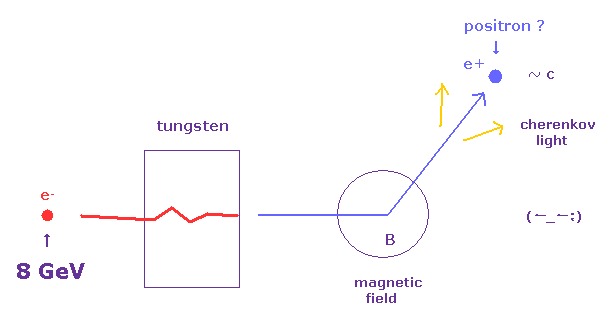In this paper, they injected very high energy electron's beam (= 8 GeV ) into tungsten target.
At the area of magnetic field, these positrons turn their moving directions by Lorentz force.

In some detection medium, high speed positrons emit cherenkov lights, which amount expresses positron's number, they insist.
But these kinds of experiments always neglect Coulomb scattering among many charged particles.

(Fig.36) Lorentz magnetic force gives only "momentum" information.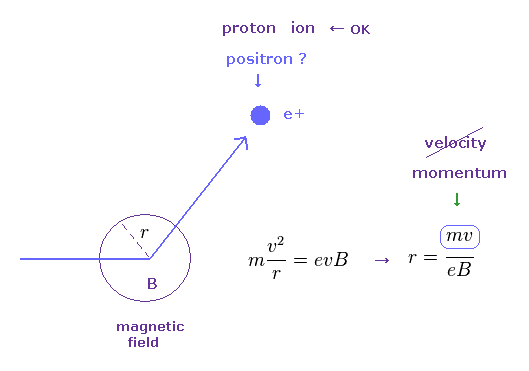In Fig.36, the centrifugal force is equal to the Lorentz magnetic force (= eBv ) under the external magnetic field (= B ).
In this case, rotating radius (= r ) is a function of momentum (= mv ).

So, we cannot distinguish positrons and other positive charges only from magnetic field.
If particles have the same momentum ( = mv ), the velocity of heavier particles becomes slower than lighter ones.

To distinguish them, they pass these particles through some radiation medium, in which only high-speed charged particles ( ex. "light" positron ) can emit cherenkov lights.
So can we detect positron correctly by this method ?

(Fig.37) They "neglect" multi-particle Coulomb scattering.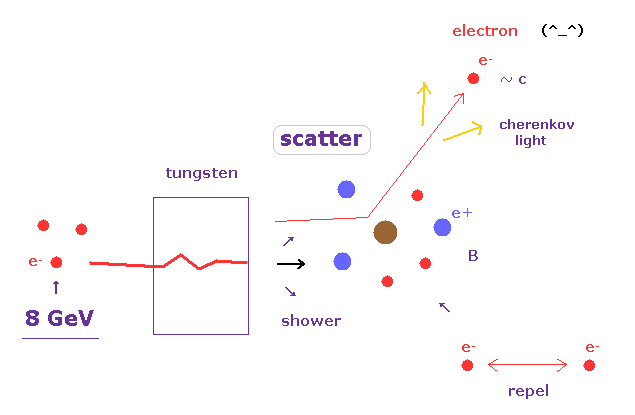But these intepretations are based on very ideal preconditions.
If there are ONLY one positron inside this device, this positron turns its moving direction by Lorentz magnetic force.

But in this case, this path is filled with a lot of charged particles such as electrons, protons, and broken nuclei.
So incident high energy electrons are easily scattered by other particles.

Or secondary electrons generated from inner wall or ions can fly into detector.
8 GeV is much bigger than electron's mc2 (= 0.5 MeV ), so ejected or scatterd electrons are high speed, too.

(Fig.38) Coulomb scattering is influential.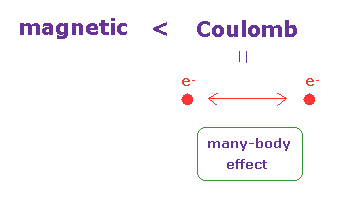Basically, researchers in the high energy accelerators tend to neglect many-body Coulomb effect.
When charged particles come near each other, Coulomb force becomes much bigger than magnetic force.

Of course, it is impossible to predict many body Coulomb effect correctly in the space filled with infinite high-speed particles.
This is the most important weakness in the high energy physics.

(Fig.39) A small number of antiparticles easily causes "error".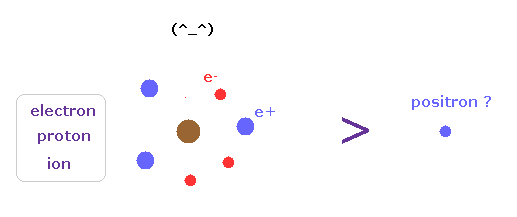Besically, the numebr of antiparticles are very small, comparing electrons, protons and ions.
So we easily mistake other charged particles for antiparticles.

## Experimental results of LHC are very doubtful.

(Fig.40) ATLAS detector consists of tracker, calorimeter, muon chamber.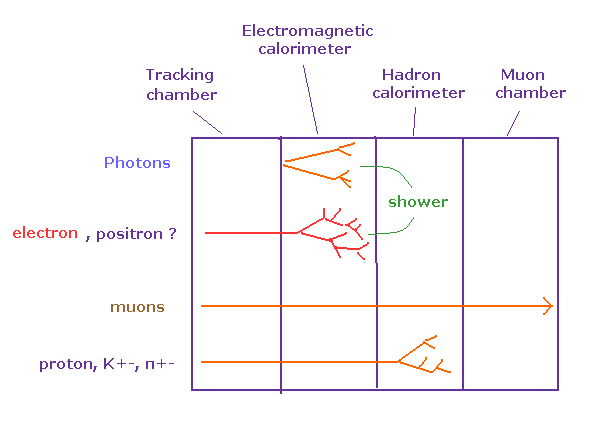As shown on this site, Detectors in accelerators consist of four major components.
In the tracking chamger, charged particles ionize electrons in the medium, which gives momentum information under magnetic field.

In the calorimeter, chared particles collide with materials and generate particle shower.
By measuring the total amount of secondary electric currents or lights, they can estimate the energy of the particle.

According to the current particle physics, muons can pass through calorimeter without stopping.
So the muon spectrometer covers the outer most layer of ATLAS detector.

(Fig.41) Silicon tracker.   Coulomb scattering obstructs Lorentz magnetic force.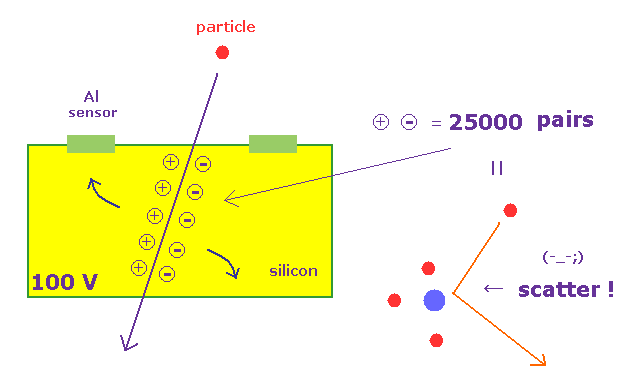The innermost part of ATLAS consists of silicon semi-conductor tracker, which can determine the position of particles, as shown on this site.
When charged particle passes through this detector, it creates ionization of electrons and holes in the bulk of silicon.

This hole drifts toward Al strips and their electric current is detected.
As shown on this site (p.4) and this site (p.40), as many as 25000 hole-electron pairs are produced, when one charged particle passes.

So, each particle is clearly scattered in these silicon detectos by Coulomb force.
This badly influences momentum measurement under magnetic field.

(Fig.42) Coulomb scattering is much greater than Lorentz magnetic force.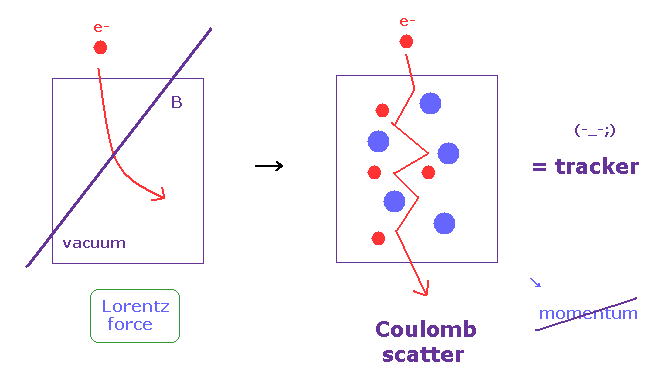As far as I see, researchers in the accelerators clearly neglect these Coulomb scattering.
Of course, it is impossible to consider all multi-Coulomb effects among infinite particles.

The measurement of particle's momentum in the tracker is very important for identifying particles.
Each particle has to pass more than 4 layers of the above silicon detectors.

So 25000 × 4 = 100000 electron-hole pairs are generated only by one particle !
Of course, each particle loses its energy, which cannot be detected correctly (= one of missing energy ).

(Fig.43) Transition radiation tracker in ATLAS.Furthermore, as shown on this site (p.23, p.25 ), each particle has to pass through 30 TRT (= transition radiation tracker ).
TRT is composed of carbon and tungsten straws with a diameter of 4 mm.

The space between the straws is filled with polypropylene materials where electrons radiate X rays.
This X rays is absorbed by Xe gas, leading to the production of a large amount of charge.

As you see, each particle has to pass various solids ( NOT vacuum ) for the measurement of its momentum.
So the interpretation of gotten momentum and particle identification based on these detectors are very doubtful.

## It's impossible to collect all energies of particle shower.

(Fig.44) Electromagnetic calorimeter.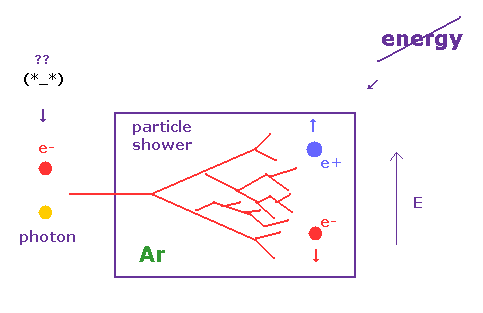As shown on this site, when photons and electrons hit the absorbers of the calorimeter, a shower of secondary particles and ionization electrons are released into liquid argon, where the presense of an electric field results in a detectable current signal.

But of course, there are some detection threshold, so all generated particles cannot be detected.
Furthermore, we cannot know kinetic energy of each secondary particle, which can give wrong missing energies.

(Fig.45)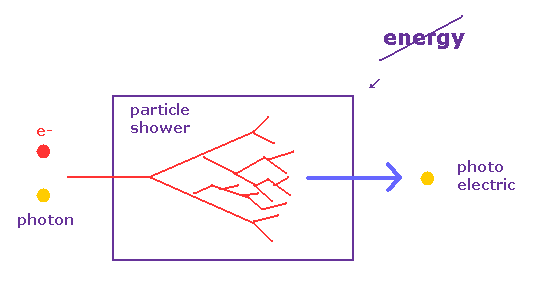When a charged particle transverses these materials, about half the lost energy is converted into inonization and half into scintillation.
The best energy resolution would obviously be obtained by collecting both the charge and light signal.

But as shown on this site (p.39), this is rarely done because of the technical difficulties to extract light and charge in the same instrument.

(Fig.46) Each transferred kinetic energy ( and light frequencies ) cannot be known.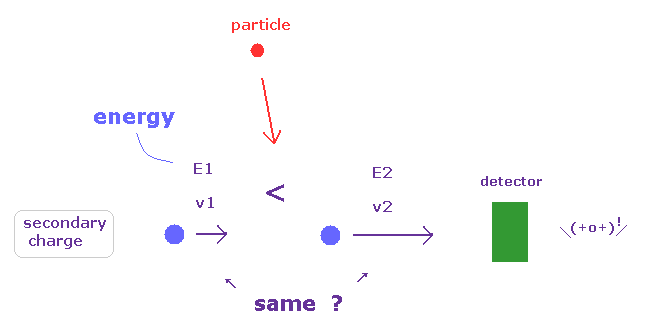The measurement of total energy in the calorimeter is based on very rough estimation.
Even if they measure total charges of secondary particles, it doesn't mean to know total energy.

Because these detectors cannot measure the kinetic energy and frequency of each particle and light.
So, the values of the missing energy (= neutrino or LSP ) gotten in the accelerators are very doubtful.

## Supersymmetry is NOT physics.

### [ Loop correction in QED is artificial trick. ]

(Fig.47) Boson and fermion's loops cancel each other ?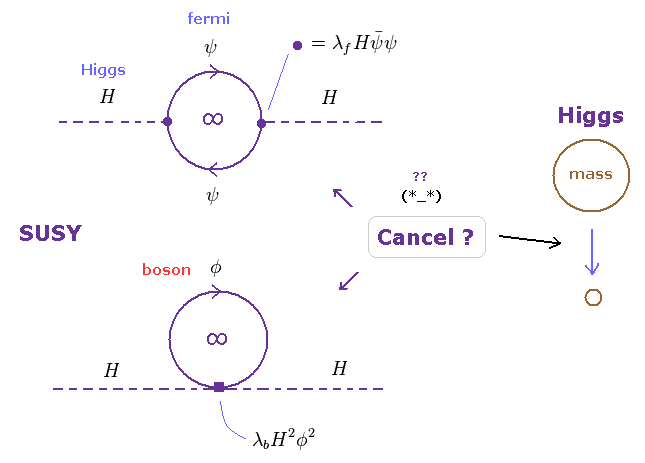As shown on this site (p.4), one loop correction of Higgs mass diverges to infinity.
At this point, you easily find the standard model based on renormalization is NOT real physics.

According to QED, fermions and bosons give infinity of the opposite sign.
This opposite sign originates in anticommutation of fermions,

Physicists NEVER try to ask what these anticommutation and infinite loop really are.
So, the standard model and supersymmetry can never advance from here.

## Chiral symmetry is abstract math ( NOT physics ) concept.

(Fig.48) "Chiral symmetry" is the reason why fermion's mass is small !?   Really ?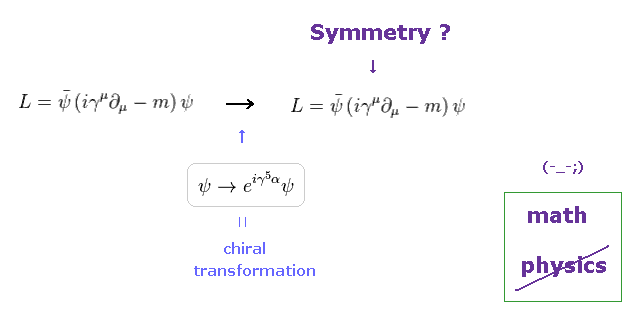Under very strict relativistic limination, all physicists could do was to define some artificial symmetry to develop their theories.
For example, in Fig.48, when Lagrangian is invariant under chiral transformation, this is called chiral symmetry.

This chiral symmetry is broken, when fermion has mass. ( So they are broken from the beggining. )
As you see, it is impossible that these abstract math symbols describe real world.

## MSSM contains "124" free parameters, "meaningless" theory.

(Fig.49) MSSM with 124 free parameters is a convenient theory.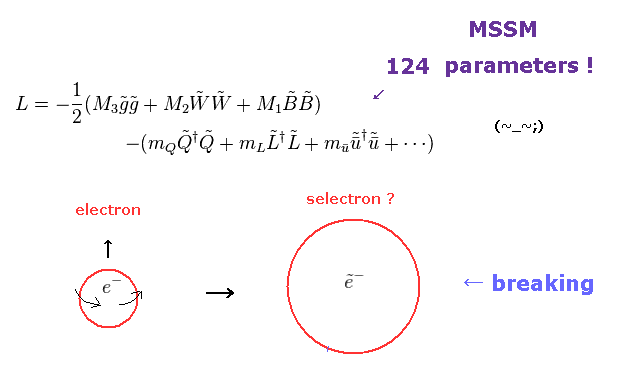The mininal supersymmetric standard model (= MSSM ) is the simplest version of SUSY.
Particle physicists tend to consider supersymmetry as most important concept.

But SUSY particles cannot be easily found. So supersymmetry is broken, which makes SUSY particle extremely heavy, they insist.
Due to this breaking, MSSM contains as many as 124 free parameters such as masses and coupling constants.

This means SUSY cannot predict anything like 10 dimensional superstring theory with 10500 vacua.
They try to waste a great deal of money and time for hunting for these meaningless SUSY.

## "Gauge symmetry" has Nothing to do with real world.

(Fig.50) "Gauge symmetry" is the reason why photon is massless ? Really ?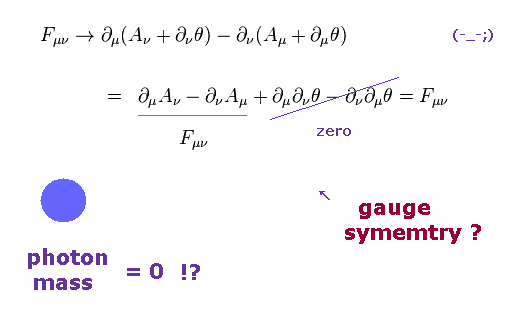In the current standard model, "gauge symmetry" is the most important principle.
When Lagrangian (= Fμν ) is invariant under gauge transformaton, this is called "gauge symmetry".

If photon has mass, this gauge symmetry is broken, so photon is massless, they insist.
But weak boson has big mass, which means this gauge symmetry is broken from the beginning, too.

So this "broken" gauge symmetry is meaningless.
They should stop blindly believing this "math" religion.

## Supersymmetry can solve fine-tuning problem of Higgs mass ?

(Fig.51) Interactive Lagrangians between Higgs and fermions ( bosons ).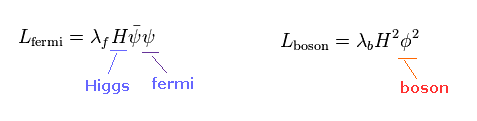As shown on this site, it is said that supersymmetry can solve fine-tuning problem in Higgs mass.
Unfortunately, these concepts are only math symbols, and have No relation with our real world.

Interactive Lagrangian between Higgs and fermions ( or bosons ) can be expressed like Fig.51.
Lagrangian (= Hamiltonian ) has very abstract form in quantum theory.

(Fig.52)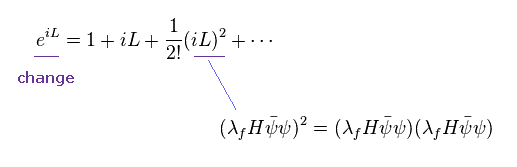Time evolution of the system obeys exponential function, which includes Lagrangian L.
In case of fermion case, quadratic term is involved in one loop divergence.

(Fig.53)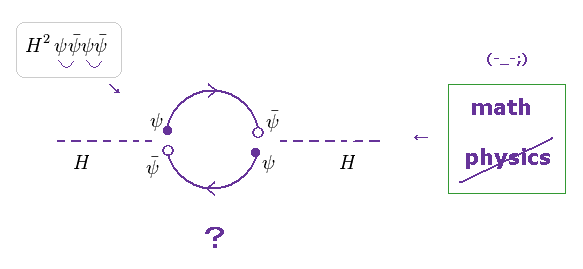Using the quadratic terms of Fig.52, Feynman diagram forms one loop, when we link each functions.
"Loop" means this equations gives infinite (= meaningless ) probability.
This is just QED math rule.

(Fig.54)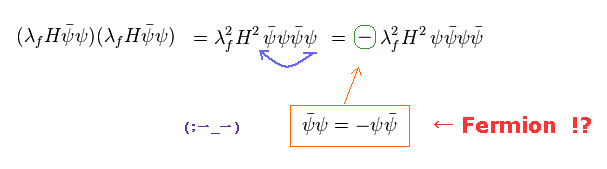In QED, the order of each function is very important.
Comparing Fig.52 and Fig.53, we need to move the right ψ to the left side.

Due to anticommutation relation of fermions, the total sign becomes opposite, when two functions are exchanged.
Of course, this is just math ( NOT physical ) reason.

To move the right ψ to the left, we have to exchange functions three times.
"Three" is odd number, so (-1)3 = -1, negative sign is added to this equation, they insist.

(Fig.55)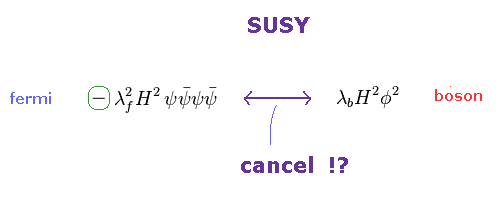On the other hand, bosons obey ordinary commutation relation.
So negative sign is NOT added, even if we exchange boson functions.

As a result, only fermion's Lagrangian becomes negative, and boson is positive.
These fermions and bosons cancel each other out, they insist.

(Fig.56)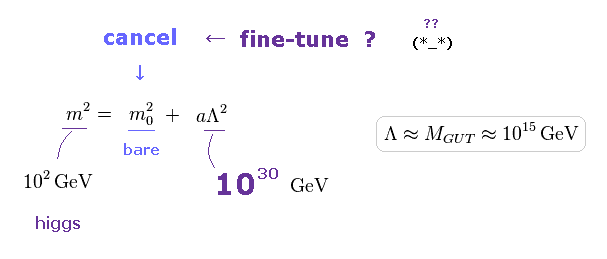If supersymmetry cannot be used, bare Higgs mass must cancel quadratic divergent term (= Λ2 ).
Quadratic divergence is as big as 1030 GeV in the grand unification theory.

Real Higgs mass is only 126 GeV, so bare Higgs mass needs to cancel as many as 30-figure values (= fine-tuning )
Unfotunately, there are NO concrete physical images here. Meaningless.

## Fermion's loop cancels boson's loop ? ← NOT physics.

(Fig.57) Fermion and boson loops cancel each other ?   "Real" physics ?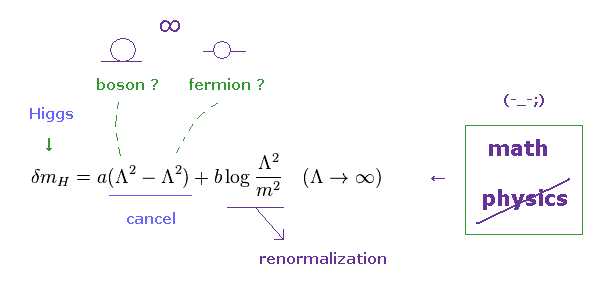If we calculate one loop divergence based on Fig.53, Fig.55 and this page, quadratic divergence is cancelled out by negative sign of fermion's loop.
So they argue supersymmetry by fermion and boson can solve fine-tuning problem of Higgs mass.

But logarithmic divergence is left, which gives infinite values.
After all, even if we introduce supersymmetry, artificial renormalization has to be used to remove infinity.

## "Chiral symmetry" is math trick,   NOT physics.

(Eq.1)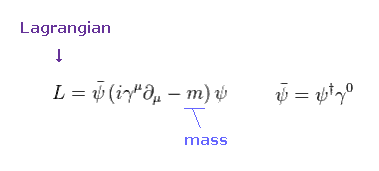In this section, we explain "chiral symmetry", which is a reason why fermion's mass is small.
Unfortunately, the concept of this symmetry is only abstract math, NOT "real" physics at all.

Lagrangian of Dirac equation can be expressed like Eq.1. See also this site (p.89).
We use "chiral expression" in 4 × 4 γ matrices, as follows,
(Eq.2)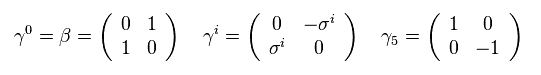(Eq.2')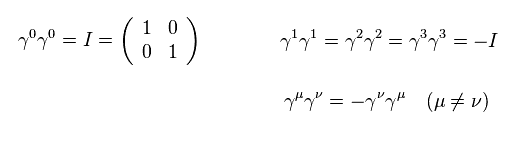If you exchange different γ matrices, negative sign is added, as shown in Eq.2'.
In Eq.2, σ is 2 × 2 Pauli matrices, as follows,
(Pa.1)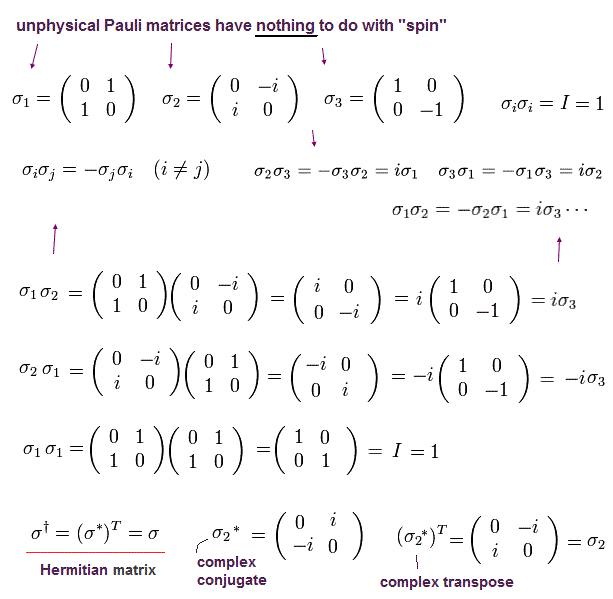Here we do the following Chiral transformation using γ5 matrix of Eq.2.
(Pa.2)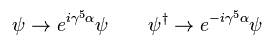If Lagrangian (= L ) remains the same under this transformation, this is called "Chiral symmetry".
So, "symmetry" means invariance of Lagrangian ( which gives Hamiltonian, equation of motion ) under some transformations such as Lorentz, gauge, supersymmetry.

(Eq.3)First, we consider the mass (= m ) of fermions is zero in Eq.3.
As a result, only the first term (= kinetic energy ) of Eq.3 is left.
(Eq.4)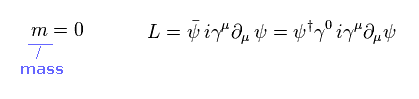"†" means complex conjugate transpose.
Under chiral transformation of Eq.3, Lagrangian of Eq.4 changes like
(Eq.5)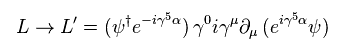From Eq.2, we have
(Eq.6)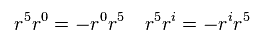From Eq.6, if γ5 moves over two (different) γ matrices, the total sign remains the same.
(Eq.7)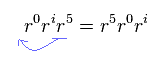As a result, Eq.5 becomes the same as the original Lagrangian ( L' = L ), as follows,
(Eq.8)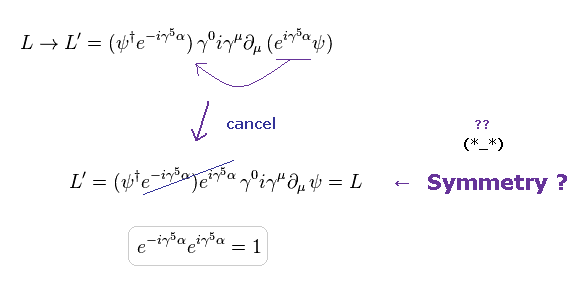Eq.8 shows when the mass of fermions is zero, its Lagnrangian remains the same under chiral transformation.
Physicists call this state "chiral symmetry", very abstract math, NOT physics.

Next, we think about the fermions having finite mass.
(Eq.9)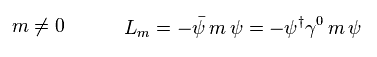The second term of Eq.3 Lagrangian is mass term (= Eq.9 ).
Under the chiral transformation, this mass term changes like
(Eq.10)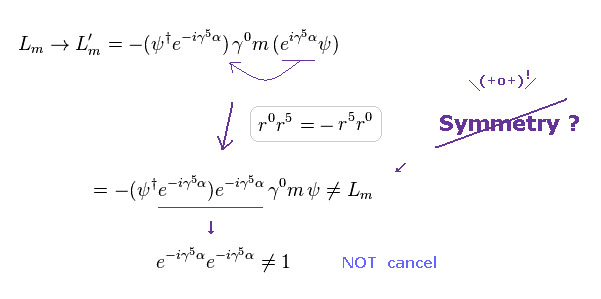As shown in Eq.10 two exponential functions don't cancel each other in this mass Lagrangian.
So they started to insist, when fermions have "mass" ( Lagrangian ), chiral symmetry is broken.

Unfortunately, there are NO physical images here.
These are just artificial math rules, do NOT mean the truth of Nature.

## "Gauge symmetry" is just math symbol, too.

(Eq.11) Gauge symmetry → photon's mass is zero !?Lagrangian of Maxwell equation contains antisymmetric tesnors (= Fμν ), as shown on this site and this site.
"Aμ" denotes vector potential, which means photon ( or W boson, gluon ).

If Lagrangian remains the same under gauge transformation (= Eq.13 ), this is called "gauge symmetry".
If photon's Lagrangian contains mass term, this Lagrangian cannot keep the same form under this transformation.

So the physicists argue, photon's mass is zero due to gauge symmetry.
Unfortunately, very abstract reason without physical reason.

(Eq.12)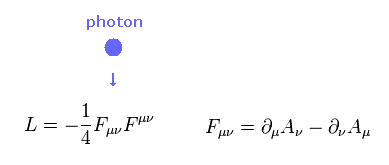(Eq.13) Gauge transformation.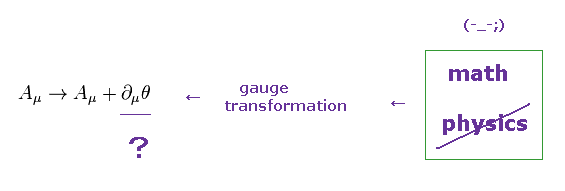Under the gauge transformation of Eq.13, Fμν of Eq.12 transforms like
(Eq.14)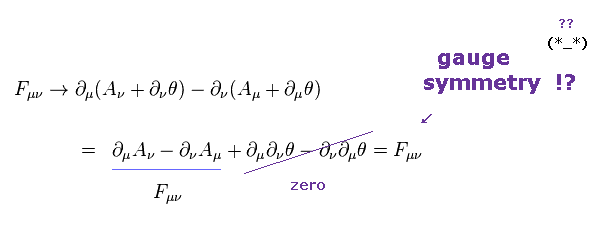As shown in Eq.14, this Fμν inside Lagrangian remains the same under this gauge transformation.
As a result, total Lagrangian remains the same, which is called "gauge symmetry".

(Eq.15) Photon's mass term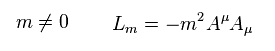Next we think about the photon's mass term inside Lagrangian.
Under the gauge transformation of Eq.13, mass Lagrangian (= Eq.15 ) tranforms like

(Eq.16)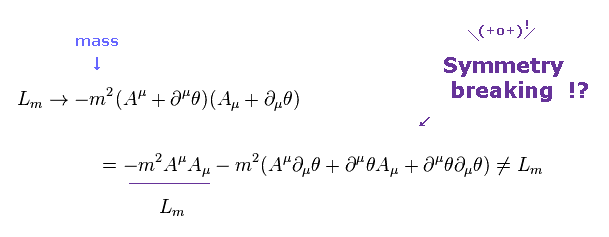As you see, mass Lagrangian of Eq.16 cannot keep its original form (= Eq.15 ).
So, physicists started to argue, photon is massless because gauge symmetry is kept.

Again, this is just abstract math reason, which has NO relation with real world.
They should clarify the true meaning of gauge (= θ ), NOT saying "Shut up and calculate !".

And W gauge boson has large mass, which means gauge symmetry is broken from the beginning.
So, this broken symmety is meaningless.

## Supersymmetry is "artificial" trick, NOT physics.

(Eq.17) Lagrangian including boson and fermion.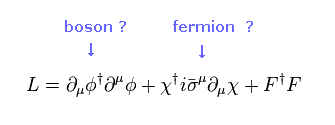If boson and fermion form "superposition" through supersymmetry, Higgs mass can be small, because the boson can utilize chiral symmetry of fermions.

Unfortunately, there are NO physical reasons at all here.
Eq.17 is Lagrangian including bosons and fermions with the same mass ( ex. m = 0 ).

(Eq.18) Supersymmetric transformation ?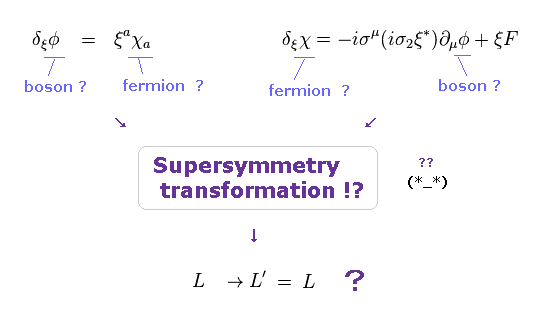As shown on this site and this site, supersymmetry transformation exchanges bosons (= φ ) and fermions (= χ ).
When Lagrangian is invariant under this transformation, this is called "supersymmetry".

As you see Eq.18, these are only artificial math rules, which do NOT represent physical reality at all.

Physicists must give up this unrealistic supersymmetry and 10-dimensional string theory in order to develop real science.
They should notice this serious fact.

## Supersymmetry is broken ← Supersymmetry is meaningless.

(Eq.19) "Broken" supersymmetry is meaningless.The important point is that we cannot easily find supersymmetric particles.
This means supersymmetry has been broken from the beginning.

So, introducing "supersymmetry" itself is meaningless.
But, the current standard model must continue creating fictitious "symmetry" to develop its theory.
This is disaster.

## Supersymmetry needs "unreal" fermions.

(Eq.20) Mass unit of bosons, fermions.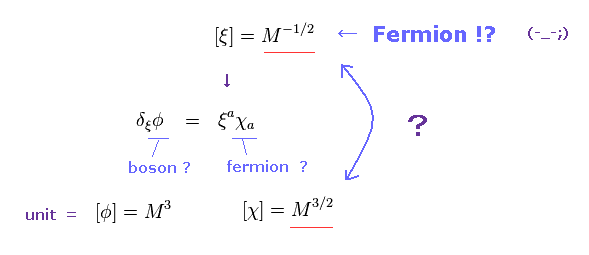Lagrangians of bosons and fermions contains different numbers of derivative, as shown in Eq.17.
So the (mass) units of boson (= φ ) and fermions (= χ ) are different.

As you notice, if boson and fermion have different units, supersymmetric transformation itself is impossible.
So theorists artificially introduced "unreal" fermions of ξ

This ξ is also fermion, but has different unit from the real fermion χ.
Of course, if there are two kinds of fermions with different units, it means self-contradiction.

As a result, the idea of supersymmetry itself is unreasonable.
But under "Shut up and calculate !" quantum theory, all physicists can do is only strange supersymmetry.
Again, this is disaster.

## Only "10-dimensional" string theory is left in supersymmetry.

(Fig.58) 26 dimensions → 10 dimensions of superstring theory !?   Fantasy ?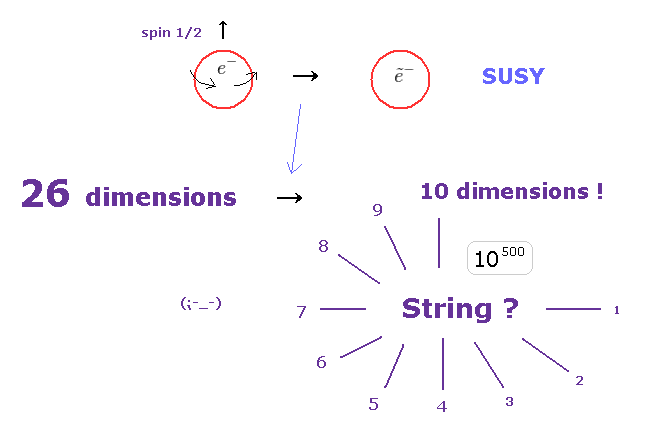As shown on this site, the original string theory consisting only of boson needs 26 spacetime dimensions !
"Super" of superstring theory means supersymmetry.

So they insist supersymmetry is indispensable for changing 26 to 10 dimensions.
Unfortunately, both these dimensions are unrealistic and fantasy.

If you ignore fatal paradox of relativity, all can you study in physics is "fantasy" string theory.2014/5/1 updated. Feel free to link to this site.